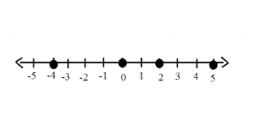# Result 5749

I think the number if I assign 8 (add) to it and divide the result by two, I get a number 46 less than the number, I guess.
What number do I think?

x =  100

### Step-by-step explanation:Did you find an error or inaccuracy? Feel free to write us. Thank you!

Tips for related online calculators
Do you have a linear equation or system of equations and looking for its solution? Or do you have a quadratic equation?